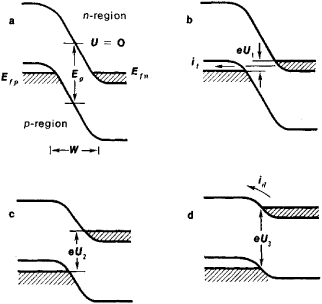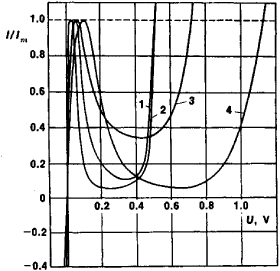# tunnel diode

(redirected from Esaki diode)
Also found in: Dictionary, Wikipedia.
Related to Esaki diode: Leo Esaki, Tunnel diode

## tunnel diode:

see diodediode
, two-terminal electronic device that permits current flow predominantly in only one direction. Most diodes are semiconductor devices; diode electron tubes are now used only for a few specialized applications.
.
The following article is from The Great Soviet Encyclopedia (1979). It might be outdated or ideologically biased.

## Tunnel Diode

(or Esaki diode), a two-terminal semiconductor electron device in which there exists an extremely thin potential barrier to electron flow (seeSEMICONDUCTOR DIODE).

The transport characteristic (the volt-ampere curve) of a tunnel diode is governed primarily by the quantum-mechanical tunneling process, which permits electrons to penetrate from one allowed energy region to the other through the barrier. The discovery of the tunnel diode in 1957 by L. Esaki provided the first convincing demonstration of electron tunneling in solids.

The construction of the tunnel diode became possible as a result of advances in semiconductor technology that permitted the< u1,="">< u2="">< u3)""="" height="304" width="322">
Figure 1. Energy diagrams for a tunnel-diode p-n junction at various bias voltages (0 < U1, < U2 < U3): (Efp) Fermi level for holes, (Efn) Fermi level for electrons, (Eg) width of energy gap, (W) width of p-n junction, (e) charge of electron, (it) tunnel current, (id) diffusion current

obtaining of semiconductor materials with sufficiently well-defined electronic properties. By doping the semiconductor with large amounts of the appropriate impurities, Esaki succeeded in making the densities of holes and electrons in the p- and n-regions, respectively, extremely high while maintaining an abrupt transition from one region to the other. If the width of the junction is very small (50–150 angstroms) and the concentration of doping agents is sufficiently high, the current through the tunnel diode is dominated by electron tunneling.

Figure 1 shows simplified energy diagrams for such p-n junctions at four different bias voltages U. As the bias is increased to the voltage U1, the interband tunnel current (it, in Figure 1,b) increases. With a further increase in the applied voltage to, say, U2 (Figure 1,c), the conduction band in the n-region becomes uncrossed with the valence band in the p-region, and the current decreases because of the lack of allowed states of corresponding energies for tunneling. In this region of the volt-ampere characteristic, the tunnel diode exhibits a negative resistance. When the voltage reaches or exceeds U3 (Figure l,d), the normal diffusion (or thermal) current dominates, as in the case of the usual p-n diode.

The first tunnel diode, which was constructed in 1957, was made of Ge. Several other semiconductor materials, however, were soon found to be suitable for obtaining tunnel diodes. Such materials include Si, InSb, GaAs, InAs, PbTe, GaSb, and SiC. Figure 2 gives the volt-ampere characteristics for tunnel diodes made from some of these materials.Figure 2. Volt-ampere characteristics of various tunnel diodes: (1) Ge diode, (2) GaSb diode, (3) Si diode, (4) GaAs diode; (U) applied bias voltage, (I/Im) ratio of current through diode and current at maximum of volt-ampere characteristic

Since the tunnel diode exhibits a differential negative resistance with a rapid response, it is capable of serving as an active element for amplification, oscillation, and switching in electronic circuits at high frequencies.

### REFERENCES

Esaki, L. “New Phenomenon in Narrow Germanium p-n Junctions.” Physical Review, 1958, vol. 109, no. 2.
Esaki, L. “Long Journey Into Tunneling.” Reviews of Modem Physics, 1974, vol. 46, no. 2.

L. ESAKI